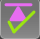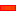﻿ MechDesigner Reference & User Interface > 2.2 Mechanism-Editor: > Force elements > Forces | Calculate Forces

# Forces | Calculate Forces

## Calculate Kinetostatic Forces Vectors

Use Calculate Forces to silently calculate Kinetostatic Forces Vectors at each joint in each kinematically-defined chain.

Calculate Forces also calculates the Application Load or Application Load Torque required from a drive system.

The correct element must be configured as the Power-Source - seeForce toolbar > Calculate Forces - is activeForces toolbar > Do Not Calculate Forces - is not active Menu : Add menu > Forces sub-menu > Calculate-Forces FB Notes: To Calculate Kinetostatic Forces Vectors: •A minimum of one Part has mass, and/or an external force is applied to the kinematic-chain with a Spring FB. See also: Show Force Vector, Tutorial 13Kinetostatic Forces - Definitions:Acceleration Definitions: